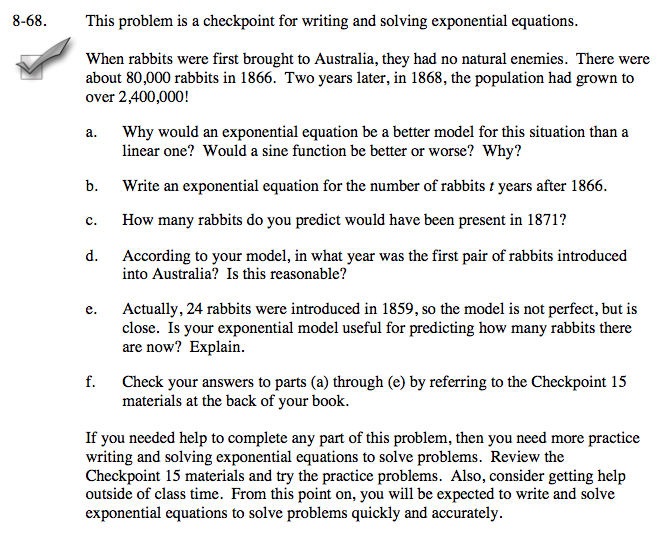### Home > A2C > Chapter 8 > Lesson 8.1.4 > Problem8-68

8-68.
1. This problem is a checkpoint for writing and solving exponential equations. Homework Help ✎When rabbits were first brought to Australia, they had no natural enemies. There were about 80,000 rabbits in 1866. Two years later, in 1868, the population had grown to over 2,400,000!

1. Why would an exponential equation be a better model for this situation than a linear one? Would a sine function be better or worse? Why?

2. Write an exponential equation for the number of rabbits t years after 1866.

3. How many rabbits do you predict would have been present in 1871?

4. According to your model, in what year was the first pair of rabbits introduced into Australia? Is this reasonable?

5. Actually, 24 rabbits were introduced in 1859, so the model is not perfect, but is close. Is your exponential model useful for predicting how many rabbits there are now? Explain.

6. Check your answers to parts (a) through (e) by referring to the Checkpoint 15 materials at the back of your book.

If you needed help to complete any part of this problem, then you need more practice writing and solving exponential equations to solve problems. Review the Checkpoint 15 materials and try the practice problems. Also, consider getting help outside of class time. From this point on, you will be expected to write and solve exponential equations to solve problems quickly and accurately.Answers and extra practice are located in the back of your printed textbook or in the Reference Tab of your eBook.
If you have an eBook for A2C , login and then click the following link: Checkpoint 15 Answers and Practice